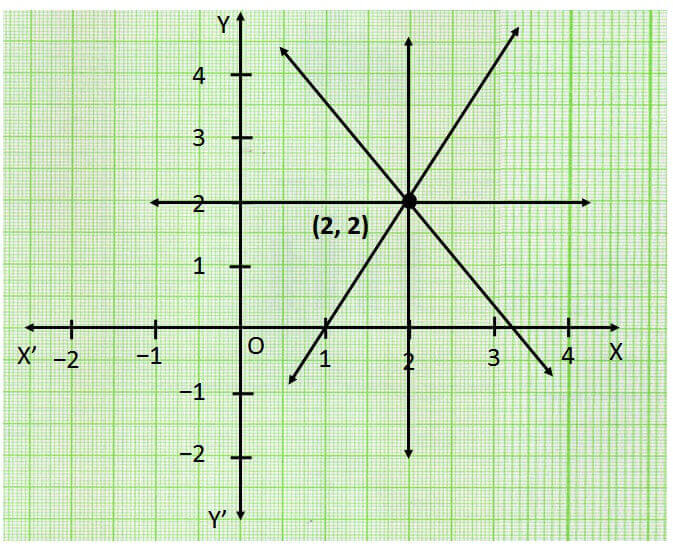Number of lines passing through a point

Chapter 4 Class 9 Linear Equations in Two Variables
Concept wise

Let us consider point (2, 2)We observe that infinite lines pass through it.

So, infinite number of line pass through a point.

Learn in your speed, with individual attention - Teachoo Maths 1-on-1 Class